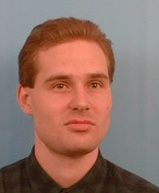Ondřej Klíma

Ph.D. thesis: Unification Modulo Associativity and Idempotency

Abstract: This thesis shows that the unification problem for the theory of one associative and idempotent binary function symbol (AI-unification), i.e. the problem of solving systems of equations in free idempotent semigroups, is NP-complete. The same result is established for the AI-matching problem, where only equations with variable-free right hand sides are considered. The complexity of the unification problem in the theory of one associative, idempotent and commutative function symbol (ACI-unification) is known to be polynomial. We describe the complexity of the unification problems for all equational theories of a binary function symbol that consist of associativity and idempotency together with any set of additional identities. We also describe the complexity of the matching problems for these theories. Unification and matching problems are solved for combinations of these theories as well. Finally, we consider the problem of solving equations from another point of view, namely, solving equations in a fixed finite idempotent semigroup. We establish almost identical dichotomy results as in the case of the unification and matching problems.

Ph.D. thesis (submitted August 2003, successfully defended March 2004) - ps-file

Extended abstract - ps-file

Refree's reports: